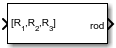# Rotation Angles to Rodrigues

Convert rotation angles to Euler-Rodrigues vector

• Library:
• Aerospace Blockset / Utilities / Axes Transformations

•## Description

The Rotation Angles to Rodrigues block converts the rotation described by the three rotation angles `R1,R2,R3` into the three-element Euler-Rodrigues vector. The rotation used in this block is a passive transformation between two coordinate systems. For more information on Euler-Rodrigues vectors, see Algorithms.

## Ports

### Input

expand all

Rotation angles, in radians, from which to determine the Euler-Rodrigues vector. Values must be double.

### Output

expand all

Euler-Rodrigues vector determined from rotation angles.

Data Types: `double`

## Parameters

expand all

Rotation order for three wind rotation angles.

The default limitations for the `'ZYX'`, `'ZXY'`, `'YXZ'`, `'YZX'`, `'XYZ'`, and `'XZY'` sequences generate an R2 angle that lies between ±pi/2 radians (± 90 degrees), and R1 and R3 angles that lie between ±pi radians (±180 degrees).

The default limitations for the `'ZYZ'`, `'ZXZ'`, `'YXY'`, `'YZY'`, `'XYX'`, and `'XZX'` sequences generate an R2 angle that lies between 0 and pi radians (180 degrees), and R1 and R3 angles that lie between ±pi (±180 degrees).

Rodrigues transformation is not defined for rotation angles equal to ±pi radians (±180 deg).

#### Programmatic Use

 Block Parameter: `rotationOrder` Type: character vector Values: `'ZYX'` | `'ZYZ'` |`'ZXY'` | `'ZXZ'` | `'YXZ'` | `'YXY'` | `'YZX'` | `'YZY'` | `'XYZ'` | `'XYX'` | `'XZY'` | `'XZX'` Default: `'ZYX'`

## Algorithms

An Euler-Rodrigues vector $\stackrel{⇀}{b}$ represents a rotation by integrating a direction cosine of a rotation axis with the tangent of half the rotation angle as follows:

`$\stackrel{\to }{b}=\left[\begin{array}{ccc}{b}_{x}& {b}_{y}& {b}_{z}\end{array}\right]$`

where:

`$\begin{array}{l}{b}_{x}=\mathrm{tan}\left(\frac{1}{2}\theta \right){s}_{x},\\ {b}_{y}=\mathrm{tan}\left(\frac{1}{2}\theta \right){s}_{y},\\ {b}_{z}=\mathrm{tan}\left(\frac{1}{2}\theta \right){s}_{z}\end{array}$`

are the Rodrigues parameters. Vector $\stackrel{⇀}{s}$ represents a unit vector around which the rotation is performed. Due to the tangent, the rotation vector is indeterminate when the rotation angle equals ±pi radians or ±180 deg. Values can be negative or positive.

 Dai, J.S. "Euler-Rodrigues formula variations, quaternion conjugation and intrinsic connections." Mechanism and Machine Theory, 92, 144-152. Elsevier, 2015.